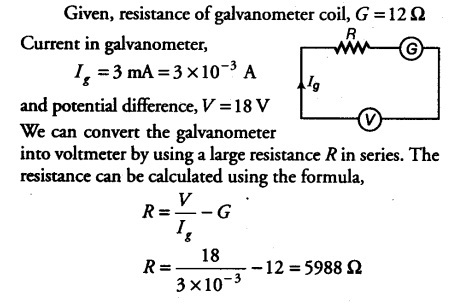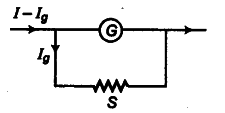# A galvanometer coil has a resistance of 12 Q

A galvanometer coil has a resistance of 12 Q and the meter shows full scale deflection for a current of 3 mA. How will you convert the meter into a voltmeter of range 0 to 18 V?This resistance {R = 5988 Ω) is connected in series with the galvanometer. The resistance is connected in series because we have to increase the resistance of the galvanometer, so that almost no current flows through it and it gives an exact value of potential difference.